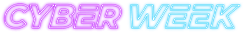#### Save big!

All courses under \$5 - for a limited time!

Code has been added to clipboard!

# Free Tutorial on JavaScript Logical Operators With Examples

Published Aug 10, 2017
Updated Oct 10, 2019

Understanding how to work with JavaScript requires logical thinking. Syntax of programming languages, be it JavaScript or any other, involves using operators that can check equality between variables and their values.

Using JavaScript logical operators lets us check equality between several variables. Now, comparison operators (such as JavaScript not equal) allow you to check the equality of JavaScript data types. In this tutorial, we will also explain what is a ternary operator JavaScript coders use and what purpose does it serve.

## JavaScript Comparison and Logical Operators: Main Tips

• JavaScript logical operators and comparison operators test for `true` or `false` values.
• Operators are used to determine differences or equality between values or variables.
• There are multiple groups of operators with different usage.

## Comparison Operators

JavaScript comparison operators are used for pairs of different variables when you need to determine their similarities and differences. The return value a comparison operator provides is of a boolean value, meaning it can be either True or False.

Given that `a = 13`, comparison operators are explained using the table below.

Operator Description Comparing Returns
== equal to a == 8 false
a == 13 true
a == "13" true
=== equal type and equal value a === 13 true
a === "13" false
!= not equal a != 8 true
!== not equal type or not equal value a !== 13 false
a !== "13" true
a !== 8 true
> greater than a > 8 true
< less than a < 8 false
>= equal to or greater than a >= 8 true
<= less than or equal to a <= 8 false

Note: notice that in JavaScript not equal type and not equal value are two different qualities!

In the example below, you can see a comparison operator being used in an `if` statement:

Example
``````if (age < 18) {
text = "Too young";
}``````

## Logical Operators

JavaScript logical operators return `true` or `false`, depending on the given information:

• JavaScript `AND` operator returns `true` only if both statements are correct.
• JavaScript `OR` operator returns `true` if one or both statements are correct. Otherwise, it returns `false`.
• JavaScript `NOT` operator returns `true` for `false` statements and vice versa.

While it might make them look similar to comparison operators, they serve a different purpose. As the name itself suggests, logical operators are used to check the logic between variables. JavaScript logical operators are mostly used in `if` statements.

The table below explains JavaScript logical operators using these example variables: `a = 9` and `b = 4`:

Operator Description Example
&& and (a < 10 && b > 1) is true
|| or (a == 5 || b == 5) is false
! not !(a == b) is true

## Conditional (Ternary) Operator

A conditional operator (`?`) selects a value based on a specified condition. It takes three operands. It is often used as a substitute for the if statement.

Operator Description
? ternary operator

In the example below, you can see how it can be used.

Example
`````` var age, voteable;
age = document.getElementById("age").value;
voteable = (age < 21) ? "You need to be 21 years old":"You are old enough";
document.getElementById("test").innerHTML = voteable + " to vote.";
``````

As you may notice in the example, using JavaScript ternary operator instead of a simple `if` statement makes the code harder to read. Therefore, make sure you don't overuse it.

## Comparing Different Types

Making JavaScript compare strings with other data types could give you an unexpected results. For example, if you are comparing a string with a number, JavaScript converts both variables to numbers and then performs the comparison:

Case Value
3 < 13 true
3 < "13" true
3 < "Billy" false
3 > "Billy" false
3 == "Billy" false
"3" < "13" false
"3" > "13" true
"3" == "13" false

If you try to make JavaScript compare strings, it will find `"3"` to be greater than `"13"`, because 1 is less than 3.

To get proper results, variables should be of the same type.

Example
``````var age, voteable;
age = Number(document.getElementById("age").value);
if (isNaN(age)) {
voteable = "Input is not a number";
}
else {
voteable = (age < 21)
? "You need to be 21 years old"
: "You are old enough";
}``````

## JavaScript Comparison and Logical Operators: Summary

• You can test for `true` or `false` values using JavaScript logical operators and comparison operators.
• You can use comparison operators to check for value and type equality.
• You can use JavaScript logical operators to check the logic between JavaScript variables and values.
• Using ternary operator JavaScript performs in the same way as it would with `if` statement.